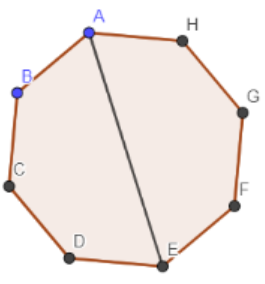What is the number of diagonals of an octagon?(a) 48(b) 40(c) 28(d) 20Verified
146.7k+ views
Hint: We know that an octagon contains 8 sides and 8 vertices and diagonal is drawn between two points. The number of diagonals is calculated by selecting two points from 8 points using combinatorial approach but selection of diagonals is done will also include 8 sides of the octagon because here we have selected all the lines that are drawn between 8 points of the octagon so we have to subtract 8 from the number of lines drawn from two points.

In the below diagram, we have drawn an octagon and also shown a diagonal between two points,In the above diagram, we have shown one of the diagonals of the octagon as AE.
In the above figure, you can see that the octagon has 8 vertices and 8 sides.
We are asked to find the number of diagonals of the octagon as any diagonal is drawn between two points so we have to select two points from 8 points to find the number of lines that can be drawn from two points.
Selecting 2 points from 8 points using combinatorial approach we get,
${}^{8}{{C}_{2}}$
We know that,
${}^{n}{{C}_{r}}=\dfrac{n!}{r!\left( n-r \right)!}$
Using the above relation to expand ${}^{8}{{C}_{2}}$ we get,
\begin{align} & {}^{8}{{C}_{2}}=\dfrac{8!}{2!\left( 8-2 \right)!} \\ & \Rightarrow {}^{8}{{C}_{2}}=\dfrac{8!}{2!\left( 6 \right)!} \\ \end{align}
We can write 8! As 8.7.6! in the above equation.
${}^{8}{{C}_{2}}=\dfrac{8.7.6!}{2!\left( 6 \right)!}$
6! Will be cancelled from numerator and denominator of the above equation we get,
\begin{align} & {}^{8}{{C}_{2}}=\dfrac{8.7}{2.1} \\ & \Rightarrow {}^{8}{{C}_{2}}=\dfrac{56}{2} \\ & \Rightarrow {}^{8}{{C}_{2}}=28 \\ \end{align}
Now, these lines from two points contain 8 lines which are the side of the octagon so we have to subtract 8 from 28 to get the number of diagonals.
\begin{align} & 28-8 \\ & =20 \\ \end{align}
Hence, the number of diagonals of the octagon is 20.
So, the correct answer is “Option D”.

Note: The mistake that could happen in the above problem is that you forgot to subtract 8 from the number of lines which are drawn between two points. The tendency of making this mistake is very high because you think that diagonals are drawn between two points in the octagon and you just select two points out of 8 points and then forget that these numbers of lines also contain the sides of the octagon.